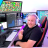# How to say set a condition of preventing shortselling in a strategy?

L

#### Lunvrsolace

##### New member
Hey guys i've been working on a HMA strategy for tos. the problem right now is is i dont know how to set a strategy condition that says you can only long as the next order after the last trade closed Or to not trigger a sell signal until there’s at least been 1 buy signal before..
I've been back testing the code to see its accuracy and i see that it keeps getting caught in short positions at the beginning of an uptrend if it has enough strength.Opened a short position right after a 'Close"Same thing here. basically want the strategy to be inactive until there's a "buy" or "avg down" after a "close"

Heres Code that can be used as a study on the 1Min Chart

Code:
``````input price = close;
input displace = 0;

input over_sold = -100;
input over_bought = 100;

def linDev = lindev(price, 1000);
def CCI = if linDev == 0 then 0 else (price - Average(price, 1000)) / linDev / 0.015;

def EMA1 = MovingAverage(AverageType.EXPONENTIAL, price, 90);
def HMA1 = MovingAverage(AverageType.HULL, price, 1800)[-displace];
def HMA2 = MovingAverage(AverageType.HULL, price, 180)[-displace];
def HMA3 = MovingAverage(AverageType.HULL, price, 40)[-displace];

def MainBullishZone = HMA1 > HMA1;
def MainBearishZone = HMA1 < HMA1;
def SubBullishZone = HMA2 > HMA2;
def SubBearishZone = HMA2 < HMA2;
def IntraBullishZone = HMA3 > HMA3;
def IntraBearishZone = HMA3 < HMA3;

plot BUYSignal = MainBullishZone and SubBearishZone and IntraBullishZone and (HMA1 < HMA2) and (HMA1 < HMA3) and (HMA3 < HMA2) and price < HMA3 and price < EMA1 and CCI < 100;

plot AvgDownSignal = MainBullishZone and SubBearishZone and intraBullishZone and price < HMA1 and price < HMA2 and CCI < 100 and HMA2 < HMA2;

plot BuyExitSignal = price crosses below HMA2 and MainBullishZone and SubBullishZone and IntraBearishZone and price > HMA1;

plot SELLSignal = MainBearishZone and SubBullishZone and IntraBearishZone and (HMA1 > HMA2) and (HMA1 > HMA3) and (HMA3 > HMA2)and price < HMA3 and price > EMA1 and CCI > -100;

AvgDownSignal.SetpaintingStrategy(PaintingStrategy.BOOLEAN_ARROW_UP);
AvgDownSignal.SetDefaultColor(Color.DARK_GREEN);

SELLSignal.SetPaintingStrategy(PaintingStrategy.BOOLEAN_ARROW_DOWN);
SELLSignal.SetDefaultColor(Color.RED);``````

Heres the code to be used as the strategy on the 1Min Chart

Code:
``````##### Betsy #####
#CONSERVATIVE SETTINGS*
# MainLength = 1800
# Sensitivity = 6

#AGGRESSIVE SETTINGS*
# MainLength = 1000
# Sensitivity = 15

input price = close;
input MainLength = 1000;
input Sensitivity = 15;
input SellAmount = 1;
input SellHeavyAmount = 35;
input AvgDownAmount = 20;

def linDev = LinDev(price, 1000);
def CCI = if linDev == 0 then 0 else (price - Average(price, 1000)) / linDev / 0.015;
def CCIDrop = CCI > CCI;

def EMA1 = MovingAverage(AverageType.EXPONENTIAL, price, 90);
def HMA1 = MovingAverage(AverageType.HULL, price, MainLength);
def HMA2 = MovingAverage(AverageType.HULL, price, 180);
def HMA3 = MovingAverage(AverageType.HULL, price, 40);

def MainBullishZone = HMA1 > HMA1[Sensitivity];
def MainBearishZone = HMA1 < HMA1[Sensitivity] and CCIDrop;
def SubBullishZone = HMA2 > HMA2;
def SubBearishZone = HMA2 < HMA2;
def IntraBullishZone = HMA3 > HMA3;
def IntraBearishZone = HMA3 < HMA3;

def BUYSignal = MainBullishZone and SubBearishZone and IntraBullishZone and (HMA1 < HMA2) and (HMA1 < HMA3) and (HMA3 < HMA2) and price < HMA3 and price < EMA1 and CCI < 100;

def AvgDownSignal = MainBullishZone and SubBearishZone and IntraBullishZone and price < HMA1 and price < HMA2 and CCI < 100 and HMA2 < HMA2;

def BuyExitSignal = price crosses below HMA2 and MainBullishZone and SubBullishZone and IntraBearishZone and price > HMA1 and CCI < 100;

def SELLSignal = MainBearishZone and SubBullishZone and IntraBearishZone and (HMA1 > HMA2) and (HMA1 > HMA3) and (HMA3 > HMA2) and price < HMA3 and price > EMA1;

def EmergencySELL = SubBearishZone and CCI < -30;

def EmergencyCOVER = CCI > 5 and CCI < 100 and CCI > CCI;

def Short =  price crosses below HMA2 and MainBullishZone and SubBullishZone and IntraBearishZone and price > HMA1 and CCI > 140;

input crossingType = {default above, below};

def avg1 = MovingAverage(AverageType.EXPONENTIAL, close, 60);
def avg2 = MovingAverage(AverageType.EXPONENTIAL, close, 180);

def signal = Crosses(avg1, avg2, crossingType == crossingType.above);
def signal2 = avg1 crosses above avg2;

AddOrder(condition = MainBearishZone, name = "Close", type = OrderType.SELL_TO_CLOSE);

Last edited:
J

#### jmoney

##### New member
I'm a bit confused by the question, but it sounds like you don't want this strategy to short at all. If that is the case, you can remove the last three lines of the strategy, and change the SELL_AUTO for buy exit signal to SELL_TO_CLOSE. I hope that helpsSet Position Exit to Low/High before Entry Bar? Questions 2